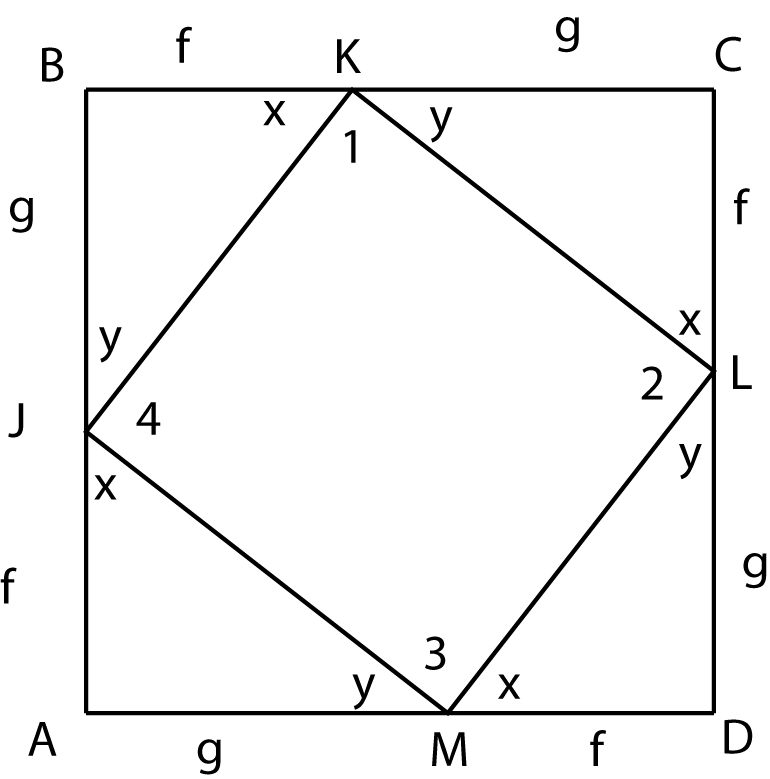### Prove: f^2 + g^2 = h^2Click or touch to draw on the diagram
Area of a square
Definition
Algebra
Algebra
Definition
CPCTC
Area postulate
SAS (Side-Angle-Side)
Algebra
Algebra
The triangle angle sum theorem
Algebra
Algebra
∠B = ∠D = ∠C = ∠A = 90
△JBK = △KCL = △LDM = △MAJ
KJ = LK = ML = JM (label them h)
∠X + ∠Y + ∠B = 180
∠X + ∠Y = 90
∠X + ∠Y + ∠1 = 180
∠1 = 90 (repeat for angles 2, 3 and 4)
KLMJ is a square
Area of ADCB = (f + g)^2
Area of ADCB = area of the four triangles + area of KLMJ
Area of ADCB = 2fg + h^2
(f+g)^2 = 2fg + h^2
f^2 + 2fg + g^2 = 2fg + h^2
f^2 + g^2 = h^2